# CHAPTER 17 - 串接氣象資料做氣象 App (1)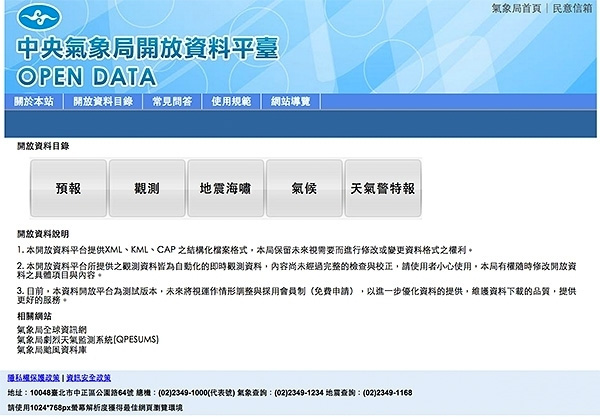``````\$(function () {
\$.ajax({
type: "GET",
url: "http://opendata.cwb.gov.tw/opendata/DIV2/O-A0001-001.xml",
dataType: "xml",
error: function (e) {
console.log('oh no');
},
success: function (e) {
var xml = e;
console.log(\$(xml));
}
});
});
``````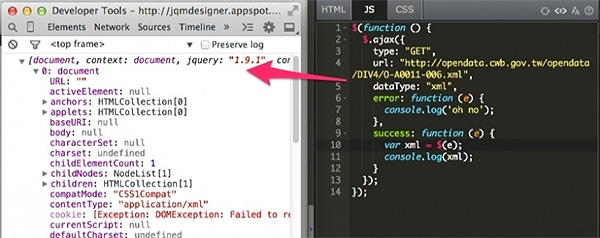``````\$(function () {
\$.ajax({
type: "GET",
url: "http://opendata.cwb.gov.tw/opendata/DIV4/O-A0011-006.xml",
dataType: "xml",
error: function (e) {
console.log('oh no');
},
success: function (e) {
var time = \$(e).find('obsTime').text();
var img = \$(e).find('uri').text();
console.log(time);
console.log(img);
}
});
});
``````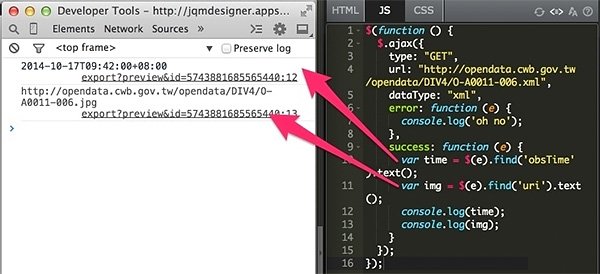HTML：

``````<div id="home" data-role="page">
<h3>南台灣雷達回波圖</h3>
</div>
<div role="main" class="ui-content">
<h4></h4>
<div class="img"></div>
</div>
</div>
``````

javascript：

``````var time = \$(e).find('obsTime').text();
var img = \$(e).find('uri').text();
\$('h4').append(time);
\$('.img').append('<img src="'+img+'"/>');
``````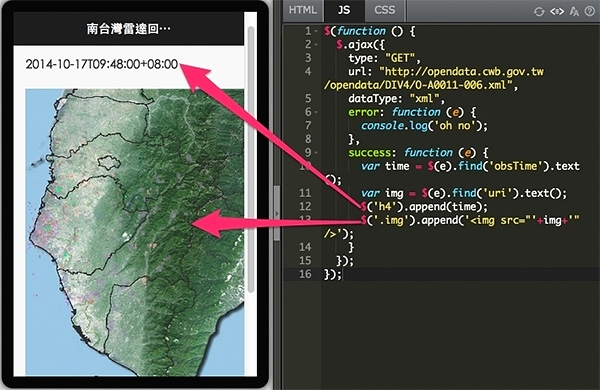``````\$(function () {
\$.ajax({
type: "GET",
url: "http://opendata.cwb.gov.tw/opendata/DIV2/O-A0001-001.xml",
dataType: "xml",
error: function (e) {
console.log('oh no');
},
success: function (e) {
var num = \$(e).find('location').length;
console.log(num);
}
});
});
``````

``````\$(function () {
\$.ajax({
type: "GET",
url: "http://opendata.cwb.gov.tw/opendata/DIV2/O-A0001-001.xml",
dataType: "xml",
error: function (e) {
console.log('oh no');
},
success: function (e) {
var data = \$(e).find('location');
var num = data.length;
var time = data.eq(0).find('time').text();
var city = data.eq(0).find('parameter').eq(0).find('parameterValue').text();
var town = data.eq(0).find('parameter').eq(2).find('parameterValue').text();
console.log(time);
console.log(city);
console.log(town);
}
});
``````HTML：

``````<div id="home" data-role="page">
<h3>台灣即時氣象</h3>
</div>
<div role="main" class="ui-content">
<li data-role="list-divider">點選城市<i class="time"></i></li>
</ul>
</div>
</div>
``````

javascript：

``````(function () {
\$(document).on("pageshow", "#home", function () {
\$.ajax({
type: "GET",
url: "http://opendata.cwb.gov.tw/opendata/DIV2/O-A0001-001.xml",
dataType: "xml",
error: function (e) {
console.log('oh no');
},
success: function (e) {
var i;
var data = \$(e).find('location');
var num = data.length;
var time = data.eq(0).find('time').text();
for (i = 0; i < num; i++) {
data.eq(i).find('parameter').eq(2).find('parameterValue').text() +
'</a></li>').listview('refresh');
}
}
});
});
})();
``````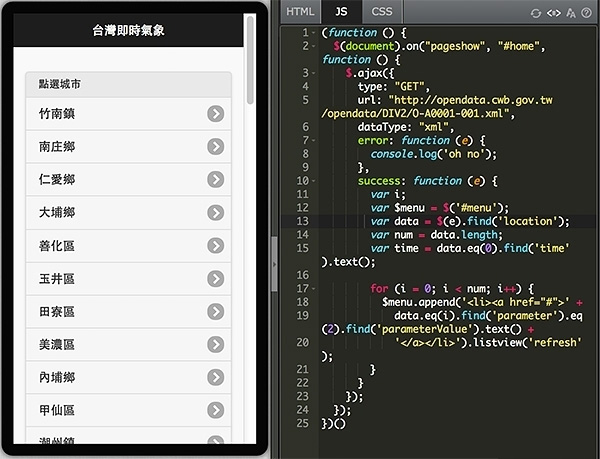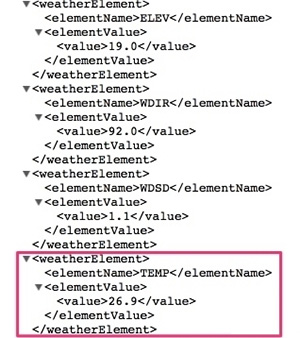HTML ( #home )：

``````<div id="home" data-role="page">
<h3>台灣即時氣象</h3>
</div>
<div role="main" class="ui-content">
<li data-role="list-divider">更新:<i class="time"></i>
</li>
</ul>
</div>
</div>
``````

HTML (#detail )：

``````<div id="detail" data-role="page" is="page">
<a class="ui-btn ui-btn-left ui-icon-arrow-l ui-btn-icon-notext" data-rel="back">Button</a>
</div>
<div role="main" class="ui-content" is="content">溫度：
<span class="temp"></span>度C
<br>濕度：
<span class="humd"></span>百分比
<br>風速：
<span class="wdsd"></span>公尺/秒
<br>日雨量：
<span class="h24r">毫米</span>
</div>
</div>
``````

javascript：

``````var temp, humd, wdsd, h24r, name;
(function () {
\$(document).on("pageshow", "#home", function () {
\$.ajax({
type: "GET",
url: "http://opendata.cwb.gov.tw/opendata/DIV2/O-A0001-001.xml",
dataType: "xml",
error: function (e) {
console.log('oh no');
},
success: function (e) {
var i,
data = \$(e).find('location'),
num = data.length,
time = data.eq(0).find('time').text();
\$('.time').append(time);

for (i = 0; i < num; i++) {
data.eq(i).find('parameter').eq(2).find('parameterValue').text() +
'</a></li>').listview('refresh');
}

\$('li a').on('click',function(){
var aIndex = \$(this).parent('li').index()-1;
temp = data.eq(aIndex).find('weatherElement').eq(3).find('elementValue').text();
humd = data.eq(aIndex).find('weatherElement').eq(4).find('elementValue').text();
h24r = data.eq(aIndex).find('weatherElement').eq(7).find('elementValue').text();
wdsd = data.eq(aIndex).find('weatherElement').eq(2).find('elementValue').text();
name = \$(this).text();
});
}
});
});
})();

(function () {
\$(document).on("pageshow", "#detail", function () {
\$('.temp').append(temp);
\$('.humd').append(humd);
\$('.h24r').append(h24r);
\$('.wdsd').append(wdsd);
\$('h3').text(name);
});
})();
``````